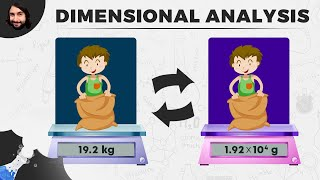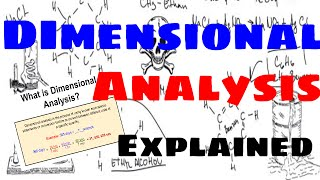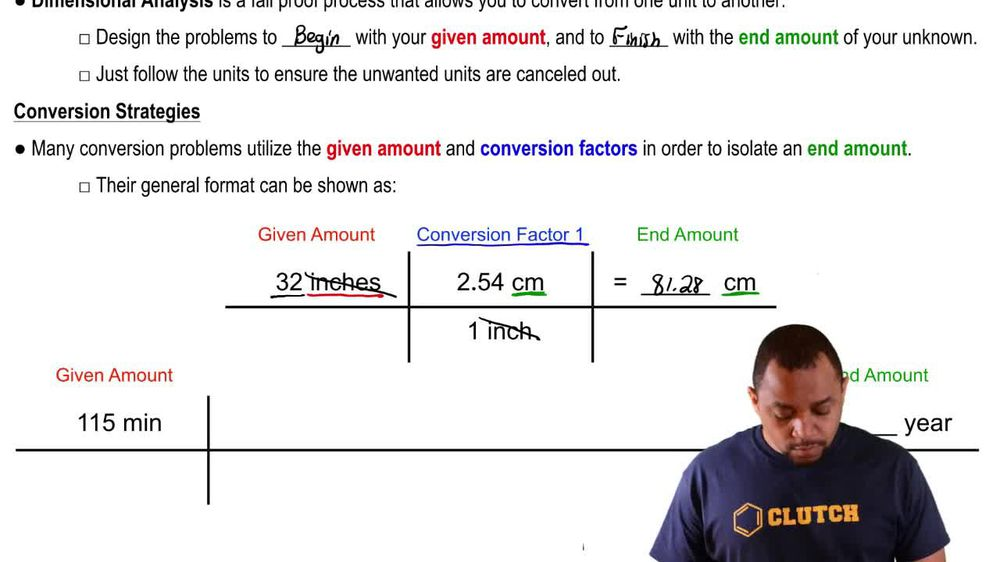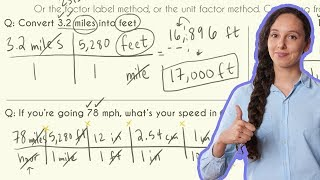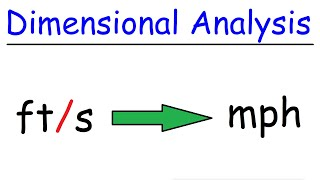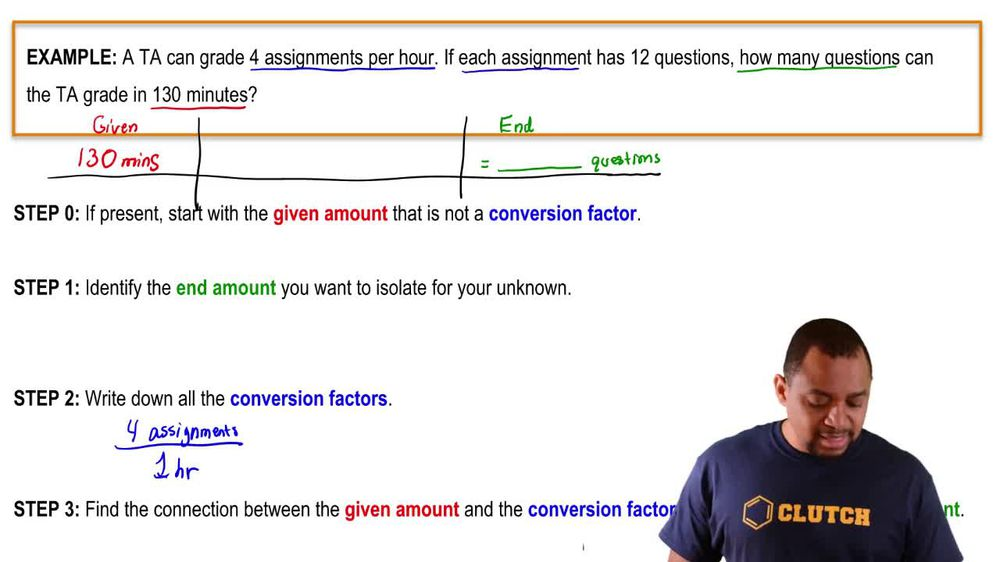Start typing, then use the up and down arrows to select an option from the list.
1. 1. Intro to General Chemistry2. Dimensional Analysis
Problem

# A period at the end of sentence written with a graphite pen-cil has a diameter of 1 mm. If the period represented the nucleus, approximately how large is the diameter of the entire atom in units of m?

Relevant Solution1m
Play a video:
Hey everyone, we're told that the tip of a pen has a diameter of five. If this represents a nucleus, what is the diameter of an entire atom in cm? To answer this question, we need to know the conversion factor of an atom diameter into a nucleus diameter. So as we've learned we have one times 10 to the negative 10 m of Adam diameter. And this is equivalent to one times 10 to the negative 15 m of nucleus diameter. Now that we have this conversion factor in mind, let's go ahead and start off with our five millimeter nucleus diameter. Now we know that we have 10-3 mm per one m. And as we stated earlier, we know that we have one times 10 to the negative 15 meters of our nucleus diameter Per one times 10 to the negative 10 m of our atom diameter. And finally we want to convert this into centimeters. So we know that we have one m and this is equivalent to 10 to the squared cm. So when we calculate this out and cancel out all of our units, We end up with a total of five times 10 to the four cm, which is going to be our final answer. Now, I hope that made sense. And let us know if you have any questions